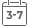# Gnc Vitamin E Ointment

GNC

Sale price \$16.46 Regular price \$24.95• G

• n

• c

• V

• i

• t

• a

• m

• i

• n

• E

• O

• i

• n

• t

• m

• e

• n

• t

• P

• r

• o

• v

• i

• d

• e

• s

• T

• e

• m

• p

• o

• r

• a

• r

• y

• R

• e

• l

• i

• e

• f

• F

• r

• o

• m

• S

• k

• i

• n

• I

• r

• r

• i

• t

• a

• t

• i

• o

• n

• s

• A

• n

• d

• R

• a

• s

• h

• e

• s

• .

• T

• h

• i

• s

• M

• o

• i

• s

• t

• u

• r

• i

• z

• i

• n

• g

• O

• i

• n

• t

• m

• e

• n

• t

• P

• r

• o

• v

• i

• d

• e

• s

• A

• P

• r

• o

• t

• e

• c

• t

• i

• v

• e

• F

• i

• l

• m

• T

• o

• S

• o

• o

• t

• h

• e

• D

• r

• y

• ,

• F

• l

• a

• k

• i

• n

• g

• S

• k

• i

• n

• .

• N

• o

• A

• r

• t

• i

• f

• i

• c

• i

• a

• l

• C

• o

• l

• o

• r

• i

• n

• g

• .

• Size : 2 oz(s)

Gnc Vitamin E Ointment Provides Temporary Relief From Skin Irritations And Rashes. This Moisturizing Ointment Provides A Protective Film To Soothe Dry, Flaking Skin. No Artificial Coloring.